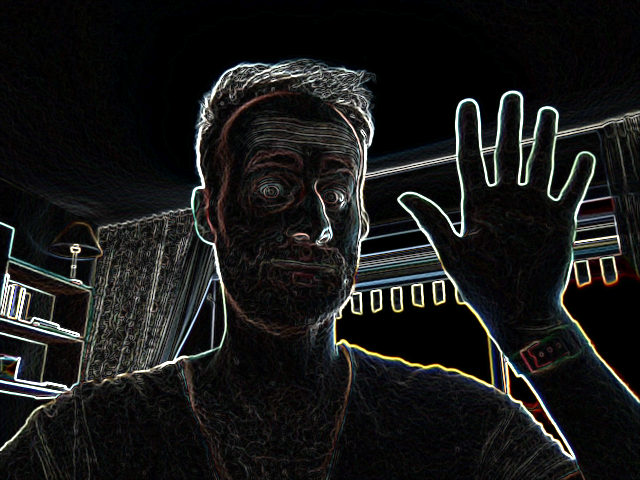# Edge detection with Sobel filters

Image processing often requires detecting edges. In this blog post I show a fragment shader that implements a “Sobel filter”, which is one method to detect edges. For a live demo, click “Start webcam” below to see edges detected in your webcam video:

What is an edge, anyway? One definition is “a steep enough gradient”. Generally, an edge detection filter differentiates a grayscale input image, and produces a grayscale output image where a pixel’s brightness in the output image corresponds to the gradient’s steepness in the input image. Take a look at what the demo does with my webcam, and consider whether “a steep gradient” really matches your intuition of what an “edge” is:In my opinion, there are some oddities. Notice the lampshade in the corner does not get a full outline. Or notice that the neckline of my T-shirt is forgotten. It doesn’t look exactly like a line drawing by a human. Nevertheless, let’s run with this definition of an edge as “a steep gradient in the image”.

Notice that the output of an edge detection filter is another grid of pixels. You may have been expecting the output to be more like a set of vectors, like an SVG. This is sometimes called “contouring” or “border following”. The demo above does not attempt to find such vectors.

Notice also that edge detection is typically defined on a “grayscale” input image. However, your webcam provides a color image. One approach is to convert the image to grayscale before detecting edges, although this throws away information (and thus edges). Another approach is to run edge detection separately on each color channel. That is what the demo above does. For example, if a line is mostly red, it means there is a steep gradient in the red color channel. Notice the strong orange line in the image above: there is little blue in it, because my blue-ish T-shirt meets the blue-ish sky in the window.

A Sobel filter is one edge detection method. It detects a gradient by performing “convolutions” on the grayscale input image. A “convolution” is a fancy name for a weighted sum of neighboring pixels. The specific weights in the sum are called a “kernel” in the jargon. Here is an example 3x3 kernel that can detect horizontal gradients (or equivalently, vertical edges):

``````1  0 -1
2  0 -2
1  0 -1
``````

For each pixel of the output, this 3x3 grid of weights is centered on the equivalent pixel in the input and its neighboring eight pixels. Each pixel is multiplied by its weight, then they’re added together to get the output.

In essence, the above kernel subtracts the brightness on the right from the brightness on the left. If all pixels are similar, the positive weights cancel with the negative weights, and the total sum is near zero. If given a horizontal gradient, from white on the left to black on the right, the kernel outputs a positive value. For example, consider a horizontal gradient that decreases by 1 for every pixel towards the right:

``````3  2  1
3  2  1
3  2  1
``````

Our Sobel filter applied to the middle pixel here gives `6`:

``````3*1  + 2*0 + 1*-1 +
3*2  + 2*0 + 1*-2 +  ==  6
3*1  + 2*0 + 1*-1
``````

If given a horizontal gradient in the other direction, from black on the left to white on the right, the kernel outputs the equivalent negative value, `-6`.

This kernel does not detect vertical gradients (or horizontal edges); it will output `0` for these. To detect vertical gradients, you can rotate the kernel to get:

`````` 1  2  1
0  0  0
-1 -2 -1
``````

But what about gradients/edges in other directions? Perhaps you can imagine designing more kernels to detect diagonal gradients. However, this is not what a Sobel filter does. Instead, a Sobel filter combines the `horizontal` and `vertical` gradients with the Euclidean distance function, `sqrt(horizontal^2 + vertical^2)`.

This is not actually equivalent to detecting a diagonal gradient! Our Sobel filter assigned a strength of `6` to horizontal and vertical gradients, but it turns out to assign a strength of `8` to an equivalent diagonal gradient. If you want to see why, consider a 45-degree gradient, from white in the top left to black in the bottom right, decreasing at the same rate of 1 per pixel. The pixel values would look like this:

``````[ 2.0*sqrt(2), 1.5*sqrt(2), 1.0*sqrt(2),
1.5*sqrt(2), 1.0*sqrt(2), 0.5*sqrt(2),
1.0*sqrt(2), 0.5*sqrt(2), 0.0*sqrt(2) ]
``````

Try applying our horizontal Sobel filter to this image; you’ll get `4*sqrt(2)`, or `5.65`, as the strength of the horizontal component of the gradient in the image. The vertical gradient would work out the same. Combining these with our distance function gives `sqrt(64)`, or `8`.

So, not perfect: a diagonal gradient is reported as 33% stronger than an orthogonal gradient. But we can get more consistent results with a different kernel. The following kernel detects a strength of 32 for both orthogonal and diagonal gradients.

``````3   0  -3
10  0  -10
3   0  -3
``````

Honestly, I don’t understand why the Sobel filter uses a 3x3 kernel. The 1x3 kernel `1 0 -1` also detects a horizontal gradient, is cheaper, works out nicely with diagonal gradients, and its output looks extremely similar, or better. If anyone knows, get in touch.

Tagged #programming, #web, #webgl. All content copyright James Fisher 2020. This post is not associated with my employer.